Question Pool Compounds of Non Metals

# Compounds of Non Metals - SAMAGRA Question Pool & Answers | Class 10 English Medium

Kerala Syllabus SAMAGRA SCERT SAMAGRA Question Pool for Class 10 English Medium Chemistry Compounds of Non MetalsQn 1.

The equation showing the reaction between potassium nitrate ( KNO3 ) and sulphuric acid (H2SO4) is givena) Complete the equation

b) Which of the given salts react with H2SO4 to form HCl

( NaNO3 , Mg(OH), CaSO4 , NaCl )

c) Write down the equation to represent the above reaction

a) HNO3

b) NaCl

c)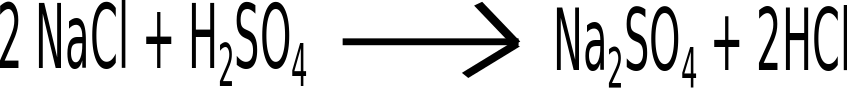Qn 2.

The equation of the reaction of Conc.H2SOwith carbon is given

0     +1+6-2                      +4 -2   +1 -2  +4 -2a) The oxydation state of which one gets increased

b) Which is the reducing agent?

c) Which substance gets reduced?

a) C

b) C

c) H2SO4

Qn 3.

When a few drops of an acid was added to blue copper sulphate crystals it was decolourised.

a) Which acid shows the above property?

b) Name the process of manufacture of the acid

c) Write any one use of the acid

a) H2SO4

b) Contact process

c) Any one use

Qn 4.

a) Choose the chemicals used to prepare ammonia from the box given below

 KNO3 , BaCl2 , NH4Cl , NaCl , Ca(OH)2, H2SO4

b) Complete the equation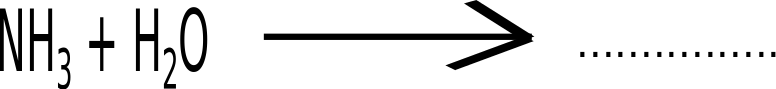c) Liquor ammonia : concentrated aquous solution of ammonia

Liquid ammonia : . .............................................

a) NH4Cl , Ca(OH)2

b) NH4OH

c) Liquified ammonia

Qn 5.

A pungent smell was felt when calcium hydroxide and ammonium chloride were mixed in a glass jar. The equation of the same is given below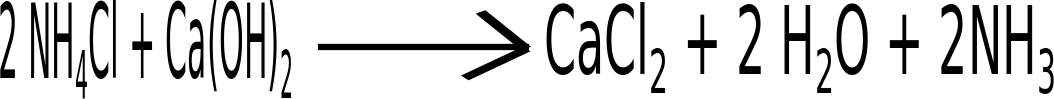a) Which is the gas formed here?

b) Write any one physical property of the gas formed

c) Write any one use of the gas formed

a) Ammonia

b) Pungent smell / dissolve in water/ Density of ammonia is less than that of air

c) For the manufacture of chemical fertilisers / as a refrigent

Qn 6.

Analyse the figure and answer the questions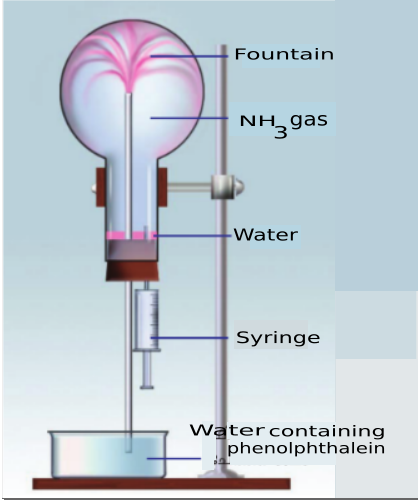a) Why did water get into the flask on pressing the piston of syringe

b) What property of ammonia is exhibited by the change of colour of water entering the flask in to pink?

c) Complete the equation(a) Decreasing the pressure in the flasks

(b) Basic nature

(c)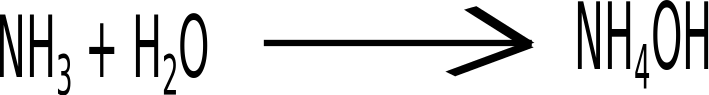Qn 7.

The figure of preparation of Ammonia in the laboratory is given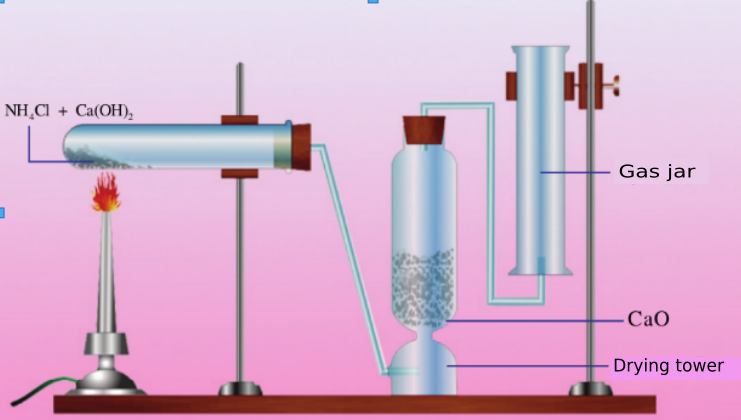a) Complete the equation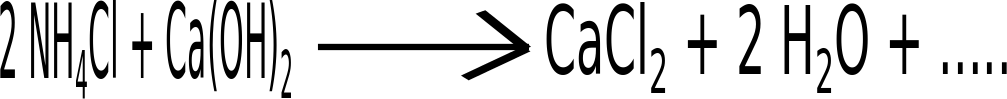b) Why is the gas formed passed through the drying tower?

c)Can sulphuric acid be used as the drying agent in ammonia preparation. Why?

d) Ammonia is collected in an inverted gas jar. Why?

a)b) To remove the moisture

c) Ammonia is a base and it reacts with sulphuric acid

d) Density of ammonia is less than that of air

Qn 8.

The equation of manufacture of ammonia is given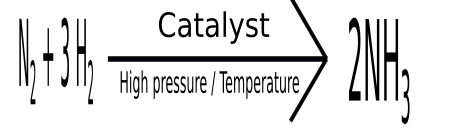a) Name the process

b) Give any one use of ammonia

c) How can you identify Ammonia

a) Haber process

b) For the manufacture of chemical fertilisers / as a refrigent

c) White fumes are formed when a glass tube dipped in HCl is  shown in ammonia gas

Qn 9.

The flow chart of manufacture of sulphuric acid is given.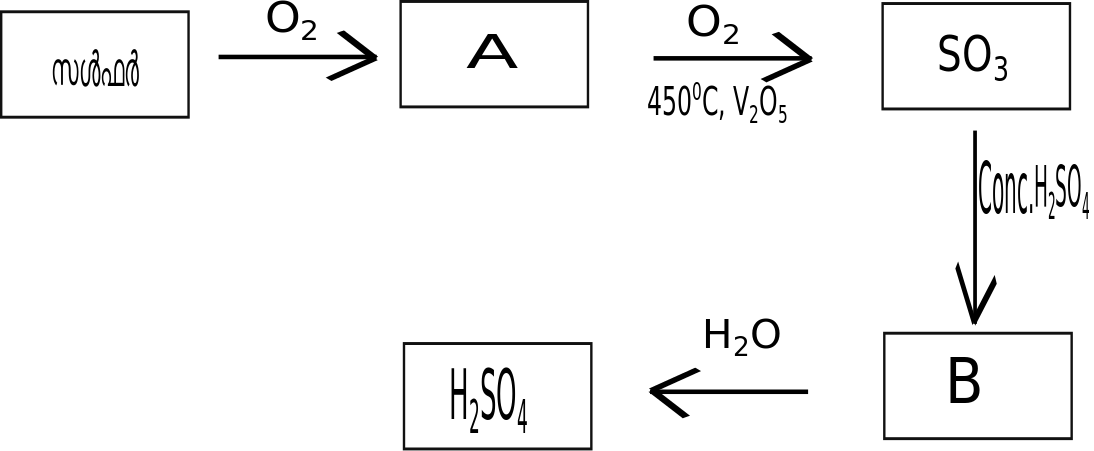a) What are A and B

b) Sulphuric acid will be formed on dissolution of SOin water. But this is not used in the manufacturing process.Why?

c) Write any one use of sulphuric acid

a) A - SO2

B - H2S2O7

b) Dissolution of SOin water is an exothermic process. So the droplets of H2SOformed causes 'fog' preventing further dissolution.

c) Any one use

Qn 10.

Different stages of manufacture of sulphuric acid are given below.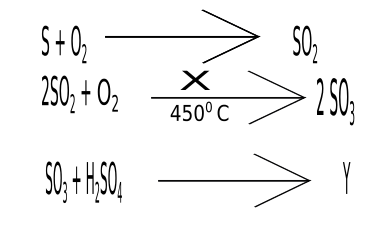a) What are X and Y

b) How is Y converted to H2SO4

c) Name the process of manufacture of Sulphuric acid

a) X - V2O5

Y - H2S2O7 ( Oleum )

b) By dissolving oleum (H2S2O7 or Y) in water.

c) Contact process

Qn 11.

Which property of sulphuric acid is exhibited in the following reactions?

I)II)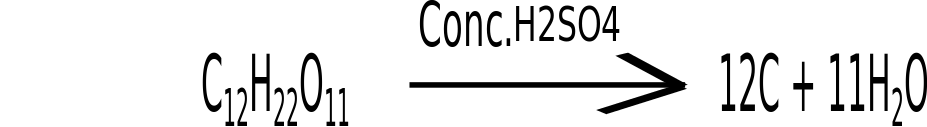a) Oxidising property

b) Dehydrating property

Qn 12.

a) A black substance is obtained when a few drops of Conc.H2SOis added to a little sugar taken in a watch glass. Identify the substance

b) Which property of sulphuric acid is exhibited here?

c) Complete the equtiona) carbon / C

b) Dehydration

c) 11 H2O

Qn 13.

Equation of the reaction between Cu and H2SOis given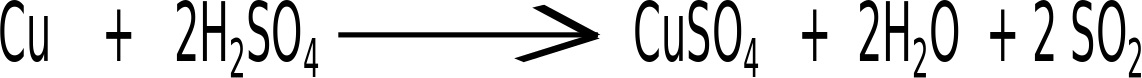a) The oxidation number of which one gets increased?

b) Which substance gets reduced?

c) Which is the reducing agent?

a) Cu

b) H2SO4

c) Cu

Qn 14.

Write down an experiment to identify sulphate salts?

 Experiment Observation Add a little barium chloride solution to the sulphate solution taken in a test tube A thick white precipitate is formed To thick white precipitate add 2-3 drops of conc.HCl white precipitate which does not dissolve in dil.HCl
Qn 15.

Complete the equation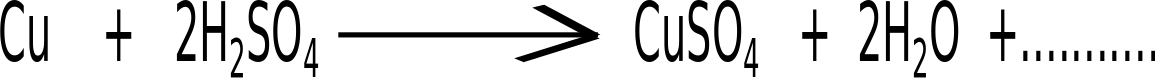b) Which is the oxidising agent in this reaction?

a) SO2

b) Sulphuric acid

Qn 16.

Equation of the reaction between Cu and H2SOis givena) The oxidation number of which one gets increased?

b) Which substance gets reduced?

c) Which is the reducing agent?

a) Cu

b) H2SO4

c) Cu

Qn 17.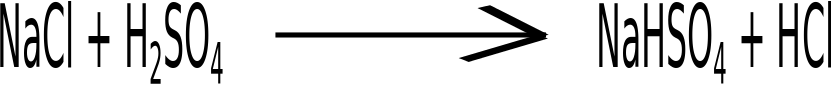a) In the above reaction sodium chloride reacts with sulphuric acid to form hydrochloric acid. Like wise, if you want to prepare nitric acid which is the salt to be used

b) Write the equation of the reaction

a) KNO3 / Any one Nitrate salt

b) KNO3 + H2SO4 →KHSO+ HNO3

Qn 18.

a) Gases like Cl2 , SO, HCl are passed through Conc. H2SOduring their laboratory preparation. Which property of sulphuric acid is utilised here?

b) NH3 gas is not passed through H2SOduring its lab preparation. Why?

a) Property as a drying agent

b) Ammonia which is basic reacts with sulphuric acid

Qn 19.

A few drops of Conc. H2SOare added to a little sugar crystals taken in a watch glass

a) What will be the observation?

b) Analyse the equation and explain the reason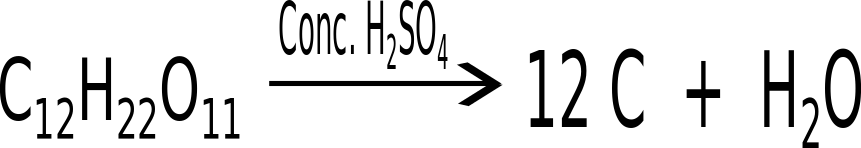c) Which property of Sulphuric acid is exhibited here?

a) Black/Brown colour develops

b) Sulphuric acid absorbs the elements hydrogen and oxygen present in sugar in the ratio 2:1 after converting it into water. So the sugar gets charred.

(or is converted to carbon)

c) Dehydration

Qn 20.

Why SOis dissolved in concentrated sulphuric acid instead of in water during the manufacture of sulphuric acid?

Dissolution of SO3 in water is an exothermic process. So the droplets of sulphuric acid formed first forms fog which prevents the further dissolution

Qn 21.a) In the above reaction sodium chloride reacts with sulphuric acid to form hydrochloric acid. Like wise, if you want to prepare nitric acid which is the salt to be used

b) Write the equation of the reaction

a) KNO3 / Any one Nitrate salt

b) KNO3 + H2SO4 →KHSO+ HNO3

Qn 22.

Complete the following equations related with the manufacture of sulphuric acid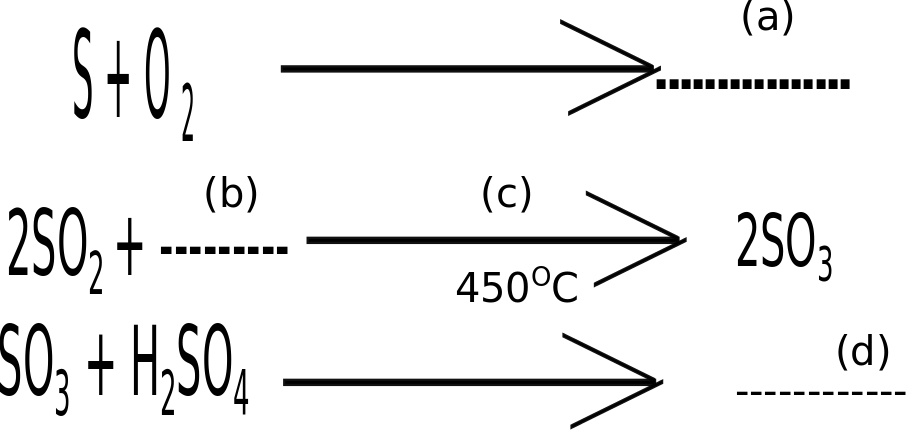i) Write a,b,c,d

ii) How is sulphuric acid prepared from oleum

i) a - SO2

b - O2

d - H2S2O7

ii) Oleum dissolved in water

Qn 23.

a) Name the process of manufacture of sulphuric acid ?

b) Which is the catalyst used in this process ?

a) Contact process

Qn 24.

Carboxylic acid + Alcohol‍ + Heat ? Ester‍ + Water

a. In order to get ester we have to heat the mixture. Do you agree with this statement. Explain your answer based on Le- Chatelier’s Principle

b. What will happen to the forward reaction if the water formed in the system is removed from this system

a. As the forward reaction is endothermic, heating leads to the formation of more amount of product

b. Rate of forward reaction increases

Qn 25.

H2 + I2 ? 2HI + Heat

Consider the equilibrium and answer the questions

a. Which reaction is favoured on increasing the concentration of I2

b. What is the effect of pressure on this equilibrium

a. Forward reaction

b. Pressure has no effect

c. High temperatures favour the backward reaction, decomposition of HI. So it is to be kept at low temperature to prevent dissociation

Qn 26.

N2O4(g)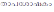?    2NO2(g)

Consider this equilibrium and complete the table given below

 Activity Change in rate of forward reaction Heats Increase the pressure Removes NO2

a. Increases

b. Decreases

c. Increases

Qn 27.

Equation showing the decomposition of calcium cabonate is given.

CaCO3(s) + Heat ? CaO(g) + CO2(g)

Say whether high temperature or low temperature is preferrable to enhance the rate of forward reaction

High temperature. As the forward reaction is endothermic, high temperature enhances the rate of forward reaction.

Qn 28.

Equation of ammonia manufacture is given

N2+3H2 ? 2NH+ Heat

a. Name the process of manufacture of ammonia.

b. What is the effect of pressure in the equilibrium?

c. Though the forward reaction is exothermic a comparatively high temperature of 4500c is used here, why?

a. Haber process

b. Rate of forward reaction increases when the pressure is increased.

c. As the forward reaction is exothermic a low temperature can be preferred. But at low temperature, the speed of forward reaction will be low as the number of molecules possessing threshold energy is less. So optimum temperature of 4500c is used.

Qn 29.

N2 + O2 + Heat  ? 2NO

How does each of the factors given below affect the rate of forward reaction?

a. Decrease in temperature

b. Increase in pressure

c. Removal of NO

a. Rate of forward reaction decreases

b. Does not have any effect in this reaction

c. Rate of forward reaction increases

Qn 30.

The graph representing a reversible reaction is given.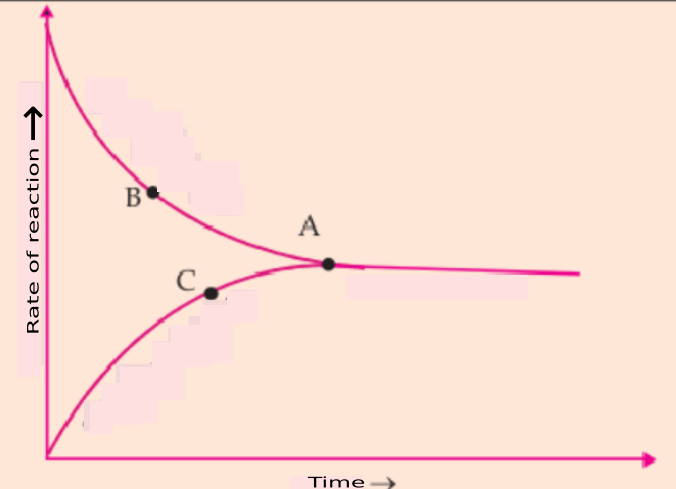a. Which of the graph represents backward reaction?

b. At which point does the system attain equilibrium?

c. When a system attains equilibrium, the concentration of reactants and products will not change. Why?

a. AC

b. A

c. Rate of forward and backward reactions are equal

Qn 31.

CO(g) + H2(g) ? CO2(g) + H2(g)

How do the factors given below affect the above system at equilibrium.

a Carbon dioxide is removed

a. Rate of forward reaction is increased

b. Rate of forward reaction is increased

c Rate of forward reaction is decreased

Qn 32.

N2(g) + 3H2(g) ? 2NH3 (g)

Consider the system at equilibrium

a. Write any two methods to increase the amount of product.

b. Which is the catalyst that can be used here?

c. What is the effect of a catalyst on an equilibrium?

a. Any two methods

b. Iron

c. A catalyst increase simultaneously the rate of forward and backward reactions, so the system can attain equilibrium very fast.

Qn 33.

2SO2(g) + O2(g) ? 2SO3(g) + Heat

a. How will the increase in the amount of oxygen affect the forward reaction?

b. Will an increase in pressure help formation of more amount of products.

a. Rate of forward reaction increases

b. Yes. According to Le- Chateleir principle, at high pressure, the system tries to decrease the volume by decreasing the number of gaseous molecules. Rate of forward reaction increases.

Qn 34.

Consider the following chemical equilibrium

14. 2CO(g) + O2(g) ? 2CO2(g)

a. What are the reactants

b. What will happen to the equilibrium if more oxygen is added to the system. Explain

c. What will be the effect of increase in pressure on the forward reaction

a. CO, O

b. According to the Le-Chateleir principle the system adjusts in such a way as to decrease the amount of oxygen.So the rate of forward reaction increases to form more products.

c. Increase the rate of forward reaction.

Qn 35.

N2(g) + 3H2(g) ? 2NH3(g) + ????

What will be the effect of the following factors on the system at equilibrium

a. Ammonia is removed from the system

b. Decreased the temperature

c. Decreased the pressure

a. Rate of forward reaction is increased to form more amount of product

b. Rate of forward reaction is increased

c. Increase the rate of backward reaction

d. Increase the rate of forward reaction

Qn 36.

A,B and C are three gases .1 mole of A reacts reversibly with 1 mole of B to form 2 mole of C.

a. Write the equation of the above reaction?

b. What will be the effect of pressure on this system when it attains equilibrium

c. What will happen to the equilibrium when more of A is added to the system

d. What will happen to the system at equilibrium when the amount of C is increased

a. A + B ? 2C

b. Pressure has no effect

c. Increase the rate of forward reaction to form more amount of products.

d. Increase the rate of backward reaction.

Qn 37.

2HI(g) + Heat ? H2(g) + I2(g)

Which among the following factor does not affect the system at equlibrium. Why?

(i) Increased the concentration of reactants

(iii) Increased the temperature

(iv) Increased the pressure

Increased the pressure. As the number of gaseous reactant molecules and gaseous product molecules are the same, pressure has no effect on this equilibrium.

Qn 38.

A system at equilibrium is given

N2 (g) + 3H2 (g) ? 2NH3 (g) + Heat

(a) When does a reversible reaction attain equilibrium.

(b) What change occur in concentration of reactants and products when the system is equilibrium

(Concetration of reactant is equal to the concentration of product, Concentration of reactants and concentration of products remain as such, Concentration of product increases)

a) When the rate of forward reaction and rate of backward reaction become equal.

b) Concentration of reactants and concentration of products remain as such

Qn 39.

Which among the following does not affect the rate of chemical reaction

(Temperature, Pressure, Colour of reactants, Concentration)

Colour of reactants

Qn 40.

Optimum temperature used in the manufacture of ammonia is -----------

4500C

Qn 41.

H2(g) + I2(g) ? 2HI(g)

Which of the following does not have any effect on the equilibrium?

(Temperature ,Pressure ,Concentration)

Pressure

Qn 42.

A System at equilibrium is given

N2O4 (g) ? 2NO2 (g)

Write any two conditions which favour the formation of the NOgas

Decrease the pressure

Increase the temperature

Qn 43.

Which of the following equilibria is not affected by change in pressure? Why?

i. H2 (g) + I2 (g) ? 2HI(g)

ii. N2O4 (g) ? 2NO2 (g)